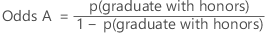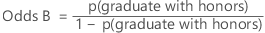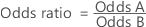# What is the odds ratio?

Use the odds ratio to compare the odds of two events. The ratio is the odds of success given that a certain condition exists divided by the odds of success given that a different condition exists. For example, you want to compare students who received home-schooling with students who attended public education. The goal of the analysis is to determine whether one group was more likely to graduate from university with honors. The odds ratio is constructed as follows:
Home-schooledPublic schoolOdds RatioThe level in the denominator is known as the reference level. In this example, Public School is the reference level. If this odds ratio equals 3.0, the odds of graduating with honors are three times greater for home-schooled students than for public school students.

You can use odds ratios by choosing the logit link function. In logistic regression, odds ratios compare the odds of each level of a categorical response variable. The ratios quantify how each predictor affects the probabilities of each response level. For example, suppose that you want to determine whether age and gender affect whether a customer chooses a hybrid car. You create a logistic regression model with the following variables:
Variable Type Description
HYBRID Binary response variable Equals 0 if the customer did not buy a hybrid car, and 1 if the customer did.
GENDER Binary predictor variable Equals 0 if the customer is male, and 1 if the customer is female. The reference level is male.
AGE Continuous predictor variable Equals the customer's age. Can equal any nonnegative value.

Suppose the logistic regression procedure declares both predictors to be significant. If GENDER has an odds ratio of 2.0, the odds of a woman buying a hybrid car are twice the odds of a man. If AGE has an odds ratio of 1.05, then the odds that a customer buys a hybrid car increase by 5% for each additional year of age.

By using this site you agree to the use of cookies for analytics and personalized content.  Read our policy